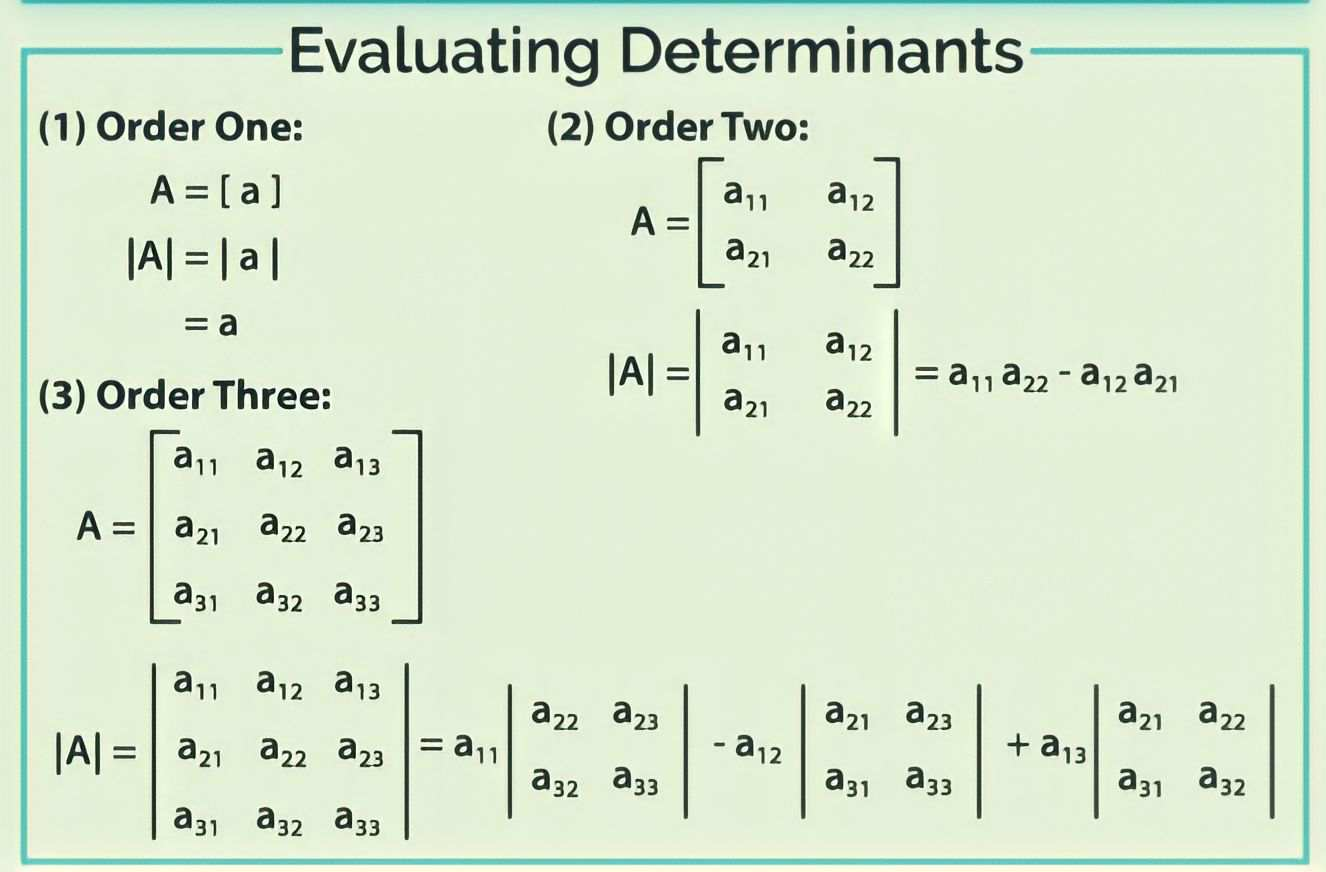# Matrices and Determinants Class 11 Mathematics Solutions | Exercise - 5.2## Chapter - 5  Matrices and Determinants

Matrices are rectangular arrays of numbers, variables, expressions or even functions which when operated upon using certain operations, can yield useful results. Determinants are scalar values that are calculated from the elements of a square matrix. It is important to understand the basics of matrices and determinants in order to solve complex algebraic equations and problems.

Before we move towards the solution section of matrices and determinant, we should learn about the topics and subtopics involved in matrices and determinants chapter. So let's have a brief introduction of the matrices and determinants chapter.

### Introduction

As mentioned before, Matrices and determinants are two related mathematical concepts used in linear algebra. A matrix is a rectangular array of numbers, symbols, or expressions arranged in rows and columns whereas determinant is a number that is derived from the elements in a matrix and it is used to describe the properties of the matrix.

Matrices and determinants chapter helps students in understanding the concept of linear equations and their solutions. It will hwlp you to learn how you can make use of matrices in solving systems of linear equations. In this chapter, we will learn about different properties of matrices and determinants, which are essential in solving problems related to matrices and determinants.

### Determinant

Determinant originally appeared in the study of linear equations. We shall, however, associate the notion of the determinant with matrices. For this purpose, we have to consider square matrices of order 2, 3 ,...,n, i.e.2×2, 3×3,... ,n×n matrices.

### Properties of Determinants

We have seen how to evaluate a determinant. Evaluation becomes easy with the help of the following properties. We shall simply verify these properties.
1. The value of the determinant is unaltered by interchanging its rows and columns.
2. Interchanging any two adjacent rows (or columns) changes the sign of the determinant.
3. If any two rows (or columns) of a determinant are identical, then the value of the determinant is zero.
4. If all the elements of any row (or column) are multiplied by a constant k, then the value of the determinant is multiplied by k.
5. If each element of any row (or column) of a determinant is expressed as the sum of two terms, then the determinant can be expressed as a sum of two determinants.
6. If two the elements of any row (or column) a multiple of any other row (or column) is added, the value of the determinant remains unaltered.### Exercise - 5.2

In this PDF, you'll only find the solution of class 11 matrices and determinants chapter. It contains all the solutions of exercise- 5.2. If you want the solutions of other exercises then you'll find them above this PDF. Just click on the button and you'll reach your destination.

NoteScroll the PDF to view all Solution

You are not allowed to post this PDF in any website or social platform without permission.

## Is Class 11 Mathematics Guide Helpful For Student ?

I have published this Notes for helping students who can't solve difficult maths problems. Student should not fully depend on this note for completing all the exercises. If you totally depend on this note and simply copy as it is then it may affect your study.

Student should also use their own will power and try to solve problems themselves. You can use this mathematics guide PDF as a reference. You should check all the answers before copying because all the answers may not be correct. There may be some minor mistakes in the note, please consider those mistakes.

## How to secure good marks in Mathematics ?

As, you may know I'm also a student. Being a student is not so easy. You have to study different subjects simultaneously. From my point of view most of the student are weak in mathematics. You can take me as an example, I am also weak in mathematics. I also face problems while solving mathematics questions.

If you want to secure good marks in mathematics then you should practise them everyday. You should once revise all the exercise which are already taught in class. When you are solving maths problems, start from easy questions that you know already. If you do so then you won't get bored.

Maths is not only about practising, especially in grade 11 you to have the basic concept of the problem. When you get the main concept of the problem then you can easily any problems in which similar concept are applied.

When your teacher tries to make the concept clear by giving examples then all students tries to remember the same example but you should never do that. You can create your own formula which you won't forget later.

If you give proper time for your practise with proper technique then you can definitely score a good marks in your examination.

Disclaimer: This website is made for educational purposes. If you find any content that belongs to you then contact us through the contact form. We will remove that content from our as soon as possible.

If you have any queries then feel free to comment down.
••